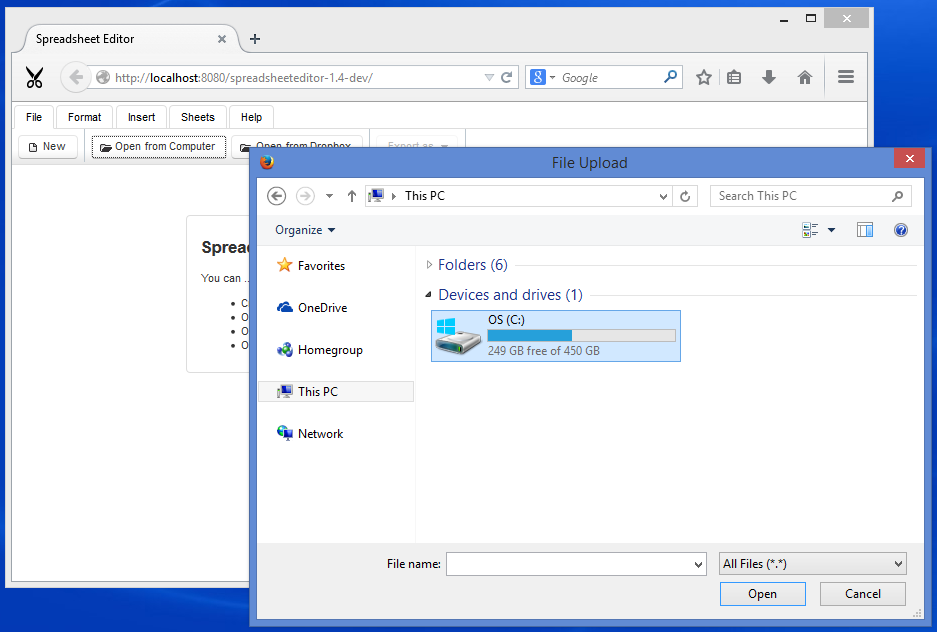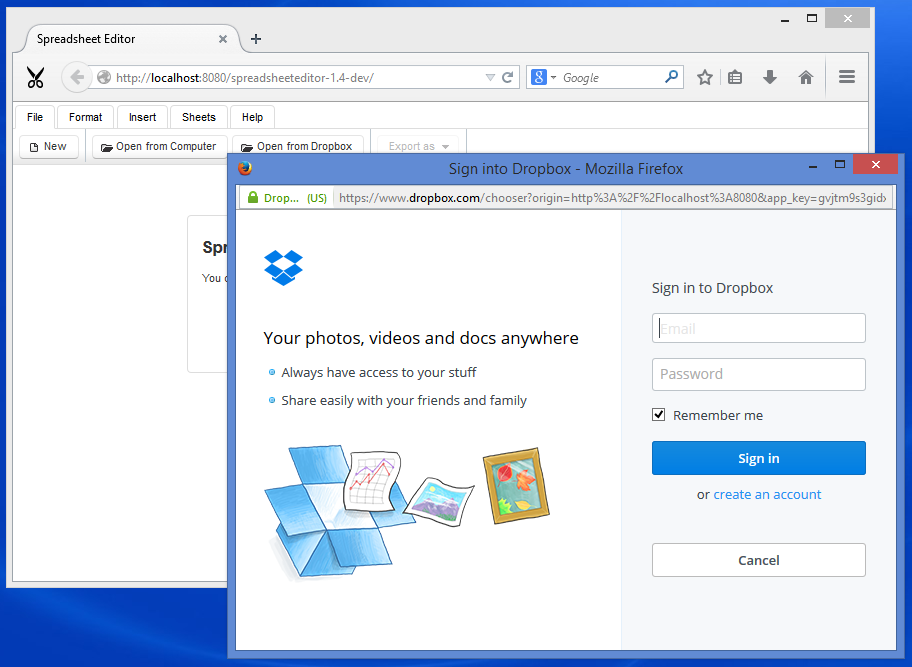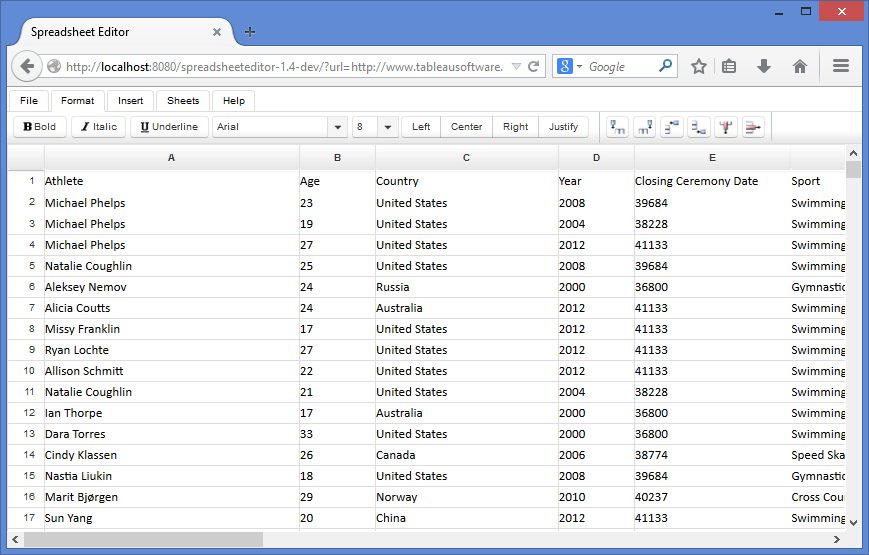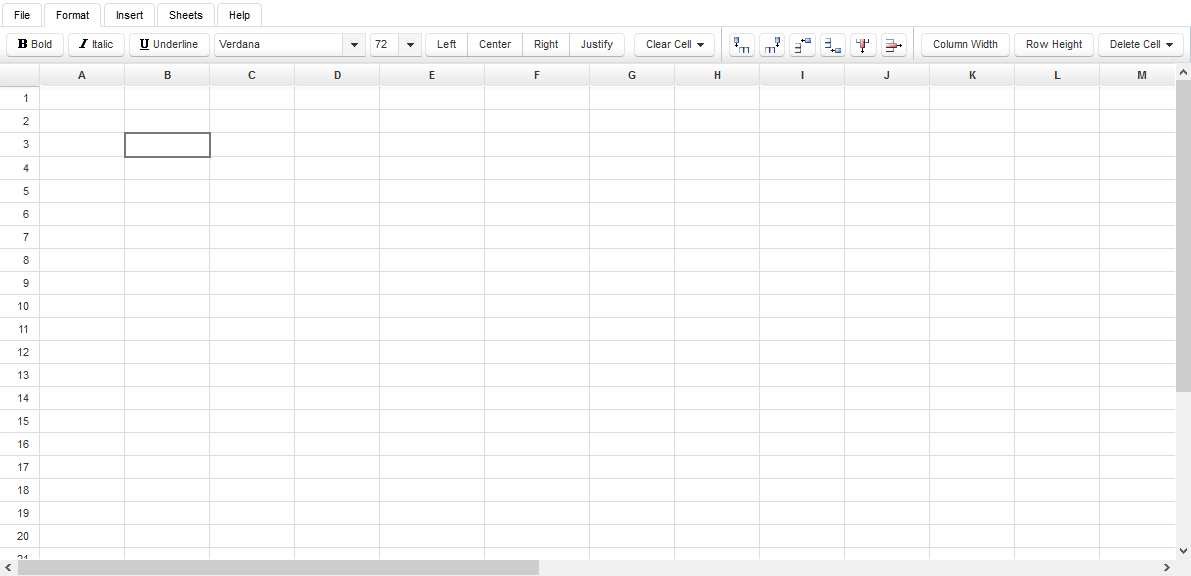# Spreadsheet Editor - Working with Files

Contents
[ ]

### Supported Files

HTML5 Spreadsheet Editor can open files in the following formats:

• Excel 1997-2003 XLS
• Excel 2007-2013 XLSX
• XLSM
• XLSB
• XLTX
• CVS
• OpenDocument

### Open Local Files

To upload a file from local computer:

1. Switch to File tab on top.
2. Click Open from Computer to open the Browse dialog.
3. Go to your desired location of file.
4. Click the desired file to select it.
5. Click Open.

The file will be opened in the editor.How it works?

The user select a file from local computer which is uploaded from web browser to server and received by PrimeFaces fileUpload component.

`` <p:fileUpload fileUploadListener="#\{workbook.onFileUpload\}" update=":ribbon :intro :sheet" />``

Managing workbook

`````` public void onFileUpload(FileUploadEvent e) {

}``````

The method LoaderService.fromInputStream reads the InputStream provided by fileUpload component create instance of com.aspose.cells.Workbook class. This instance is kept in memory as long the user keeps viewing or editing the spreadsheet in web browser. When user leaves the editor or close the browser the unused instances are automatically unloaded from memory to keep the server clean.

`````` public String fromInputStream(InputStream s, String name) {

com.aspose.cells.Workbook w;

try (InputStream i = s) {

w = new com.aspose.cells.Workbook(i);

} catch (Exception x) {

return null;

}

String key = this.generateKey();

this.workbooks.put(key, w);

this.buildCellsCache(key);

this.buildColumnWidthCache(key);

this.buildRowHeightCache(key);

return key;

}``````

Caching

Caching is very important to HTML5 Spreadsheet Editor. It makes everything work smooth. The CellsService keep cache rows, columns, cells and properties of all workbooks loaded by the editor. When LoaderService loads a spreadsheet completely, it reads the it from top to bottom and fills up the cache by calling LoaderService.buildCellsCache, LoaderService.buildColumnWidthCache, LoaderService.buildRowHeightCache

``````     public void buildCellsCache(String key) {

com.aspose.cells.Workbook wb = workbooks.get(key);

com.aspose.cells.Worksheet ws = wb.getWorksheets().get(wb.getWorksheets().getActiveSheetIndex());

int maxColumn = ws.getCells().getMaxColumn() + 1;

maxColumn = maxColumn + 26 - (maxColumn % 26);

int maxRow = 20 + ws.getCells().getMaxRow() + 1;

maxRow = maxRow + 10 - (maxRow % 10);

ArrayList<Column> columns = new ArrayList<>(maxColumn);

ArrayList<Row> rows = new ArrayList<>(maxRow);

for (int i = 0; i < maxColumn; i++) {

}

for (int i = 0; i < maxRow; i++) {

}

for (Object o : ws.getCells()) {

com.aspose.cells.Cell c = (com.aspose.cells.Cell) o;

rows.get(c.getRow()).putCell(c.getColumn(), cells.fromAsposeCell(c));

}

for (int i = 0; i < maxRow; i++) {

for (int j = 0; j < maxColumn; j++) {

String col = com.aspose.cells.CellsHelper.columnIndexToName(j);

if (!rows.get(i).getCellsMap().containsKey(col)) {

rows.get(i).putCell(col, cells.fromBlank(j, i));

}

}

}

cells.putColumns(key, columns);

cells.putRows(key, rows);

}``````

``````     public void buildColumnWidthCache(String key) {

com.aspose.cells.Workbook wb = workbooks.get(key);

com.aspose.cells.Worksheet ws = wb.getWorksheets().get(wb.getWorksheets().getActiveSheetIndex());

ArrayList<Integer> columnWidth = new ArrayList<>();

for (int i = 0; i < cells.getColumns(key).size(); i++) {

}

cells.putColumnWidth(key, columnWidth);

}``````

``````     public void buildRowHeightCache(String key) {

com.aspose.cells.Workbook wb = workbooks.get(key);

com.aspose.cells.Worksheet ws = wb.getWorksheets().get(wb.getWorksheets().getActiveSheetIndex());

ArrayList<Integer> rowHeight = new ArrayList<>();

for (int i = 0; i < cells.getRows(key).size(); i++) {

}

cells.putRowHeight(key, rowHeight);

}``````

### Open from Dropbox

To open files from Dropbox:

1. Switch to File tab on top.
2. Click Open from Dropbox to open Dropbox file chooser.
3. If you are not already signed-in, it will require you to sign-in to your Dropbox account.
4. Navigate to desired file and click to select it.
5. Click Choose at the bottom.

Your selected file will be opened from Dropbox.How it works?

The Open from Dropbox button uses Dropbox JavaScript Chooser API to open Dropbox Chooser dialog. The Chooser provides URL of selected file, which is captured by the callback function and sent back to the server. The server creates a instance of spreadsheet from URL, initialize some housekeeping stuff, and send DOM updates back to the browser. The browser renders and refresh the HTML and user is ready to edit the loaded document.

### Open from URL

Files can be directly opened from URLs. This allows user to edit any publicly available file on Internet. To open the file append ?url=location parameter with the value of your desired location while loading the editor. For example:

`` http://editor.aspose.com/?url=http://example.com/Sample.xlsx``How it works?

Instantiate during startup

When WorksheetView backend bean is instantiated by JSF the PostConstruct method init is called which loads the spreadsheet using LoaderService.fromUrl.

Caching

When spreadsheet is ready at server side, JSF components are used to generate new HTML and send DOM updates to user which are rendered by the web browser.

``````     @PostConstruct

private void init() {

String requestedSourceUrl = FacesContext.getCurrentInstance().getExternalContext().getRequestParameterMap().get("url");

if (requestedSourceUrl != null) {

try {

this.sourceUrl = new URL(requestedSourceUrl).toString();

} catch (MalformedURLException x) {

msg.sendMessageDialog("The specified URL is invalid", requestedSourceUrl);

}

}

}``````

``````     public String fromUrl(String url) {

com.aspose.cells.Workbook w;

try (InputStream i = new URL(url).openStream()) {

w = new com.aspose.cells.Workbook(i);

} catch (Exception x) {

throw new RuntimeException(x);

}

String key = generateKey();

workbooks.put(key, w);

buildCellsCache(key);

buildColumnWidthCache(key);

buildRowHeightCache(key);

return key;

}``````

``````     public void buildCellsCache(String key) {

com.aspose.cells.Workbook wb = workbooks.get(key);

com.aspose.cells.Worksheet ws = wb.getWorksheets().get(wb.getWorksheets().getActiveSheetIndex());

int maxColumn = ws.getCells().getMaxColumn() + 1;

maxColumn = maxColumn + 26 - (maxColumn % 26);

int maxRow = 20 + ws.getCells().getMaxRow() + 1;

maxRow = maxRow + 10 - (maxRow % 10);

ArrayList<Column> columns = new ArrayList<>(maxColumn);

ArrayList<Row> rows = new ArrayList<>(maxRow);

for (int i = 0; i < maxColumn; i++) {

}

for (int i = 0; i < maxRow; i++) {

}

for (Object o : ws.getCells()) {

com.aspose.cells.Cell c = (com.aspose.cells.Cell) o;

rows.get(c.getRow()).putCell(c.getColumn(), cells.fromAsposeCell(c));

}

for (int i = 0; i < maxRow; i++) {

for (int j = 0; j < maxColumn; j++) {

String col = com.aspose.cells.CellsHelper.columnIndexToName(j);

if (!rows.get(i).getCellsMap().containsKey(col)) {

rows.get(i).putCell(col, cells.fromBlank(j, i));

}

}

}

cells.putColumns(key, columns);

cells.putRows(key, rows);

}``````

``````     public void buildColumnWidthCache(String key) {

com.aspose.cells.Workbook wb = workbooks.get(key);

com.aspose.cells.Worksheet ws = wb.getWorksheets().get(wb.getWorksheets().getActiveSheetIndex());

ArrayList<Integer> columnWidth = new ArrayList<>();

for (int i = 0; i < cells.getColumns(key).size(); i++) {

}

cells.putColumnWidth(key, columnWidth);

}``````

``````     public void buildRowHeightCache(String key) {

com.aspose.cells.Workbook wb = workbooks.get(key);

com.aspose.cells.Worksheet ws = wb.getWorksheets().get(wb.getWorksheets().getActiveSheetIndex());

ArrayList<Integer> rowHeight = new ArrayList<>();

for (int i = 0; i < cells.getRows(key).size(); i++) {

}

cells.putRowHeight(key, rowHeight);

}``````

To create a new empty spreadsheet:

1. Switch to File tab.
2. Click the New button.

The editor will close the opened spreadsheet, if any, and will open a new one.How it works?

Instantiate a new object

Caching

When spreadsheet is ready at server side, JSF components are used to generate new HTML and send DOM updates to user which are rendered by the web browser.

``````     public void loadBlank() {

}``````

``````     public String fromBlank() {

com.aspose.cells.Workbook w = new com.aspose.cells.Workbook();

String key = generateKey();

workbooks.put(key, w);

buildCellsCache(key);

buildColumnWidthCache(key);

buildRowHeightCache(key);

return key;

}``````

#### buildCellsCache

``````     public void buildCellsCache(String key) {

com.aspose.cells.Workbook wb = workbooks.get(key);

com.aspose.cells.Worksheet ws = wb.getWorksheets().get(wb.getWorksheets().getActiveSheetIndex());

int maxColumn = ws.getCells().getMaxColumn() + 1;

maxColumn = maxColumn + 26 - (maxColumn % 26);

int maxRow = 20 + ws.getCells().getMaxRow() + 1;

maxRow = maxRow + 10 - (maxRow % 10);

ArrayList<Column> columns = new ArrayList<>(maxColumn);

ArrayList<Row> rows = new ArrayList<>(maxRow);

for (int i = 0; i < maxColumn; i++) {

}

for (int i = 0; i < maxRow; i++) {

}

for (Object o : ws.getCells()) {

com.aspose.cells.Cell c = (com.aspose.cells.Cell) o;

rows.get(c.getRow()).putCell(c.getColumn(), cells.fromAsposeCell(c));

}

for (int i = 0; i < maxRow; i++) {

for (int j = 0; j < maxColumn; j++) {

String col = com.aspose.cells.CellsHelper.columnIndexToName(j);

if (!rows.get(i).getCellsMap().containsKey(col)) {

rows.get(i).putCell(col, cells.fromBlank(j, i));

}

}

}

cells.putColumns(key, columns);

cells.putRows(key, rows);

}``````

#### buildColumnWidthCache

``````     public void buildColumnWidthCache(String key) {

com.aspose.cells.Workbook wb = workbooks.get(key);

com.aspose.cells.Worksheet ws = wb.getWorksheets().get(wb.getWorksheets().getActiveSheetIndex());

ArrayList<Integer> columnWidth = new ArrayList<>();

for (int i = 0; i < cells.getColumns(key).size(); i++) {

}

cells.putColumnWidth(key, columnWidth);

}``````

#### buildRowHeightCache

``````     public void buildRowHeightCache(String key) {

com.aspose.cells.Workbook wb = workbooks.get(key);

com.aspose.cells.Worksheet ws = wb.getWorksheets().get(wb.getWorksheets().getActiveSheetIndex());

ArrayList<Integer> rowHeight = new ArrayList<>();

for (int i = 0; i < cells.getRows(key).size(); i++) {

}

cells.putRowHeight(key, rowHeight);

}``````

### Export to Various Formats

After editing files, the user will want to save changes. The editor allows user to export and download the modified spreadsheet to local computer. To export the file:

1. Switch to File tab on top.
2. Click Export as button.
3. Choose your desired format from the dropdown.

The modified file will be exported for download. The following formats are supported for export:

• Excel 2007-2013 XLSX
• Excel 1997-2003 XLS
• Excel XLSM
• Excel XLSB
• Excel XLTX
• Excel XLTM
• Portable Document Format (PDF)

How it works?

The opened spreadsheet is converted to user-specified format using WorksheetView.getOutputFile.

``````     public StreamedContent getOutputFile(int saveFormat) {

byte[] buf;

String ext = null;

switch (saveFormat) {

case com.aspose.cells.SaveFormat.EXCEL_97_TO_2003:

ext = "xls";

break;

case com.aspose.cells.SaveFormat.XLSX:

ext = "xlsx";

break;

case com.aspose.cells.SaveFormat.XLSM:

ext = "xlsm";

break;

case com.aspose.cells.SaveFormat.XLSB:

ext = "xlsb";

break;

case com.aspose.cells.SaveFormat.XLTX:

ext = "xltx";

break;

case com.aspose.cells.SaveFormat.XLTM:

ext = "xltm";

break;

ext = "xml";

break;

case com.aspose.cells.SaveFormat.PDF:

ext = "pdf";

break;

case com.aspose.cells.SaveFormat.ODS:

ext = "ods";

break;

}

try {

ByteArrayOutputStream out = new ByteArrayOutputStream();

getAsposeWorkbook().save(out, saveFormat);

buf = out.toByteArray();

} catch (Exception x) {

throw new RuntimeException(x);

}

return new DefaultStreamedContent(new ByteArrayInputStream(buf), "application/octet-stream", "Spreadsheet." + ext);

}``````